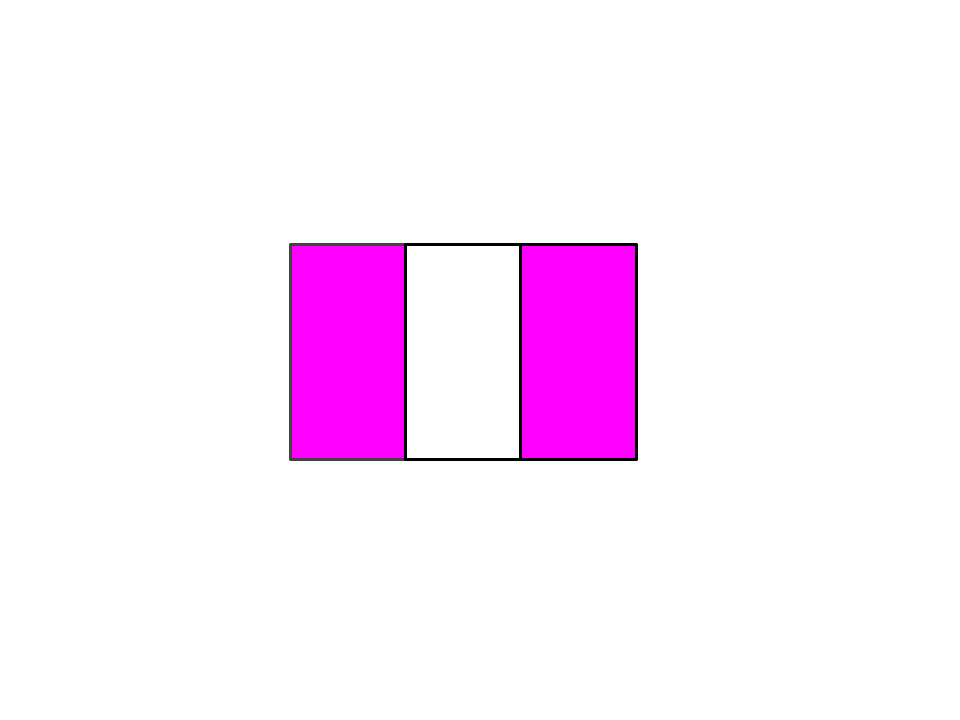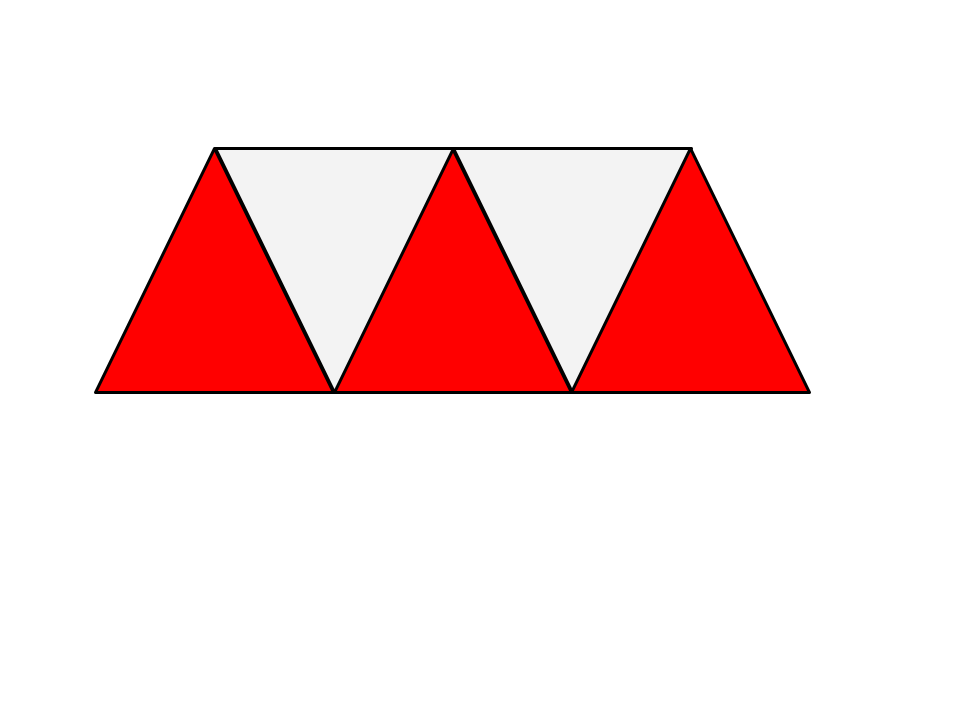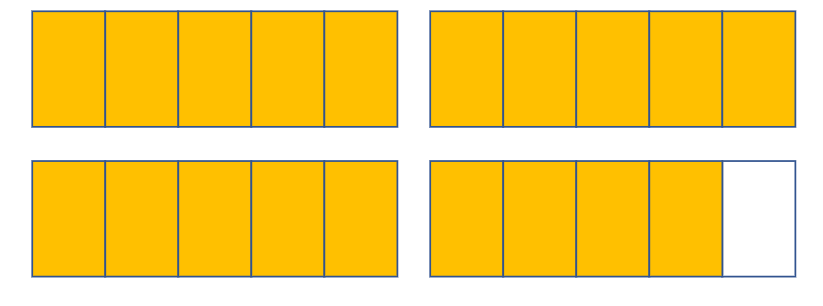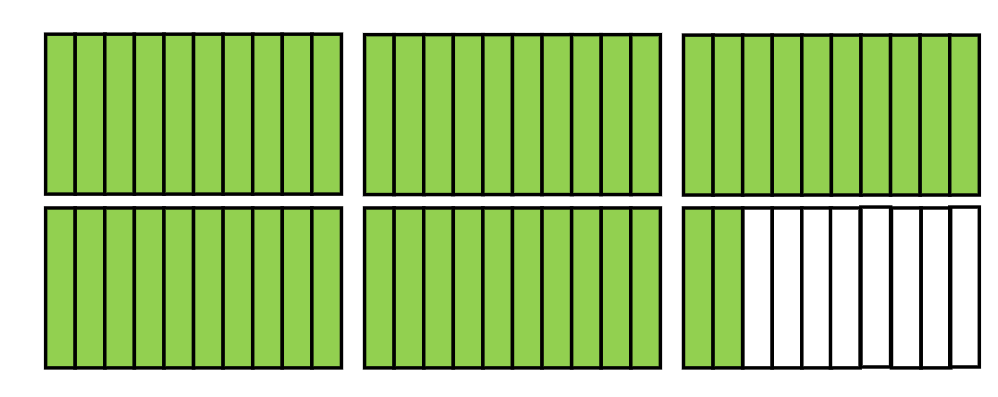Identifying fractions
Compare and Order Fractions
Subtracting Fractions
Multiplying & Dividing Fractions
Fractions & Vocab
100

What fraction of the star is shaded?1/2

100

Compare the fractions using <, >, or =

5/6     4/6

5/6  >  4/6

100

Add the fractions:   2/5  +  3/5

1 OR 5/5

100

Subtract the fractions:  19/20  -  6/20

13/20

100

Multiply the fractions:   4/7 x 1/7

4/49

100

What is the top number of a fraction called?

Numerator

200

What fraction of the rectangle is shaded pink?2/3

200

Compare the fractions using <, >, or =.

3/4     3/8

3/4  >  3/5

200

11/20

200

Subtract the fractions:   4/3 - 1/7

25/21

200

Multiply the fractions:   3/7 x 5/10

15/70

200

What is the bottom number in a fraction called?

Denominator

300

What fraction of the shape is red?3/5

300

Compare the fractions using <, >, or =.

10/20   10/12

10/20 < 10/12

300

Add the fractions:  1/4 + 3/8

5/8

300

Subtract the fractions:  2 2/7 -  1  8/14

10/14

300

Divide the fractions:   4/7 / 3/8

32/21

300

What does the fraction bar in the middle of a fraction mean?

Out of or divided by

4003 4/5

400

Compare the fractions using <, >, or =.

5/8     1/2

>

400

3 1/8   +    5 6/16

8 8/16

400

8/9 -  2/3

2/9

400

Multiply:    2/6   x   5/3

10/18

400

What vocabulary word means the same as equal?

Equivalent

5005 2/10

500

Compare the fractions using >, <, =

2/10     20/100

2/10 = 20/100

500

3 8/10 + 2 2/5 =

6 2/10

500

4 4/10  - 1 4/5

2 6/10  Or  2   3/5

500
Divide:  2/10   /    3/12

24/30

500

Name a way fractions can be used in real life?

to divide food, money or other items

Click to zoom